Processing ......FreeComputerBooks.com Links to Free Computer, Mathematics, Technical Books all over the World

Analyzing US Census Data: Methods, Maps, and Models in R
Top Free Machine Learning Books 🌠 - 100% Free or Open Source!
• Title: Analyzing US Census Data: Methods, Maps, and Models in R
• Author(s) Kyle Walker
• Publisher: Chapman and Hall/CRC; 1st edition (February 16, 2023); eBook (Creative Commons Licensed)
• Hardcover: 378 pages
• eBook: PDF and ePub
• Language: English
• ISBN-10/ASIN: 1032366443
• ISBN-13: 978-1032366449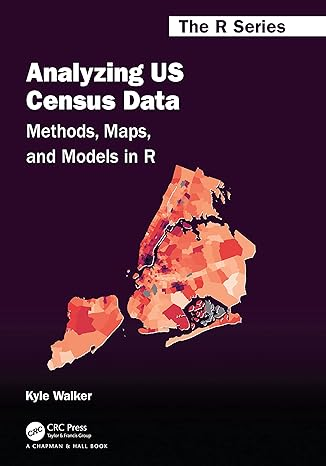Book Description

Census data is widely used by practitioners to understand demographic change, allocate resources, address inequalities, and make sound business decisions.

This book introduces readers to tools in the R programming language for accessing and analyzing Census data from the United States Census Bureau and shows how to carry out demographic analyses in a single computing environment.

• Kyle Walker is an associate professor of geography at Texas Christian University, director of TCU’s Center for Urban Studies, and a spatial data science consultant.
Reviews, Ratings, and Recommendations: Related Book Categories: Read and Download Links:Similar Books:
•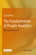The Fundamentals of People Analytics: With Applications in R

Human capital is an organization’s most important asset. Address this need by curating key concepts spanning the entire analytics lifecycle, along with step-by-step instructions for their applications to real-world problems, using open-source software.

•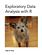Exploratory Data Analysis with R (Roger D. Peng)

This book covers the essential exploratory techniques for summarizing data with R. These techniques are typically applied before formal modeling commences and can help inform the development of more complex statistical models.

•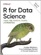R for Data Science: Visualize, Model, Transform, Tidy, Import

This book teaches you how to do data science with R: You'll learn how to get your data into R, get it into the most useful structure, transform it, visualize it and model it, how data science can help you work with the uncertainty and capture the opportunities.

•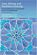Data Mining and Analysis: Fundamental Concepts and Algorithms

This textbook provides a broad yet in-depth overview of data mining, integrating related concepts from machine learning and statistics. The main parts of the book include exploratory data analysis, pattern mining, clustering, and classification.

•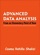Advanced Data Analysis from an Elementary Point of View

This is a textbook on data analysis methods, intended for advance undergraduate students who have already taken classes in probability, mathematical statistics, and linear regression. It presumes that you can read and write simple functions in R.

•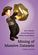Mining of Massive Datasets (Jure Leskovec, et al)

It focuses on practical algorithms that have been used to solve key problems in data mining and can be applied successfully to the largest datasets. It begins with a discussion of the map-reduce framework, an important tool for parallelizing algorithms automatically.

•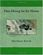Data Mining for the Masses (Matthew North)

This book uses simple examples, clear explanations and free, powerful, easy-to-use software to teach you the basics of data mining; techniques that can help you answer some of your toughest business questions.

•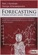Forecasting: Principles and Practice (Rob J. Hyndman, et al.)

This textbook provides a comprehensive introduction to forecasting methods and presents enough information about each method for readers to use them sensibly. Examples use R with many data sets taken from the authors' own consulting experience.

•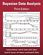Bayesian Data Analysis (Andrew Gelman, et al.)

This classic book is widely considered the leading text on Bayesian methods, lauded for its accessible, practical approach to analyzing data and solving research problems. It takes an applied approach to analysis using up-to-date Bayesian methods.

•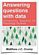Answering Questions with Data (Matthew Crump, et al.)

This is a free textbook teaching introductory statistics for undergraduates. Students will learn to select an appropriate data analysis technique, carry out the analysis, and draw appropriate conclusions.

•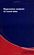Basic Data Analysis and More - A Guided Tour using Python

In this book, a selection of frequently required statistical tools will be introduced and illustrated. An exemplary implementation of the presented techniques using the Python programming language is provided.

•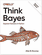O'Reilly® Think Bayes: Bayesian Statistics in Python

If you know how to program, you're ready to tackle Bayesian statistics. With this book, you'll learn how to solve statistical problems with Python code instead of mathematical formulas, using discrete probability distributions rather than continuous mathematics.

•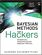Bayesian Methods for Hackers: Probabilistic Programming

This book illuminates Bayesian inference through probabilistic programming with the powerful PyMC language and the closely related Python tools NumPy, SciPy, Matplotlib, through practical examples and computation - no advanced mathematics required.

•An Introduction to Bayesian Thinking (Merlise Clyde, et al.)

This book provides an introduction to Bayesian inference in decision making without requiring calculus. It may be used on its own as an open-access introduction to Bayesian inference using R for anyone interested in learning about Bayesian statistics.

Book Categories
 :All CategoriesTop Free BooksRecent BooksMiscellaneous BooksComputer EngineeringComputer LanguagesComputer ScienceData Science/DatabasesJava and Java EE (J2EE)Linux and UnixMathematicsMicrosoft and .NETMobile ComputingNetworking and CommunicationsSoftware EngineeringSpecial TopicsWeb Programming
Other Categories